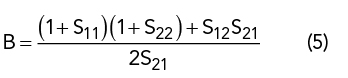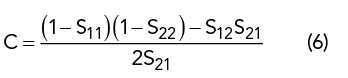Figure 1 |S21| response of a 500 MHz Butterworth lowpass filter, showing the effect of a 0.1 pF stray capacitance in parallel with the ideal inductor.

The intuitive understanding of an RF network is only possible if its behavior can at least be understood in first order terms. To aid this understanding, RLC parameter extraction can be very enlightening.

To illustrate, knowing that a 0.1 pF effective capacitance exists between the nodes of a lowpass filter might lead to a superior design: shielding between the nodes, resulting in greater stopband isolation. Figure 1 plots the response of a three element, 500 MHz Butterworth filter, showing the effect of 0.1 pF stray capacitance across an ideal inductor. Above 4 GHz, the isolation is clearly compromised by the stray capacitance.

While this simple example assumes ideal components, it is reasonable to say that stray capacitance between the nodes of a lowpass filter is detrimental - which is why high isolation, lumped-element lowpass filters have shields between sections, where the capacitors to ground are implemented with a feedthrough capacitor in the wall of each shield.

ABCD PARAMETERS

Actual components are never ideal, making mathematical extraction useful to understand their limitations. ABCD parameters, which are also known as cascade, chain or T parameters, are particularly useful for this purpose. For a two-port network, the ABCD parameters are defined as1:Figure 2 Two-port network signal flow for ABCD parameters.

where v1 and v2 are the input and output voltages, i1 flows into the network on the left side and i2 flows out of the network on the right side (see Figure 2). i2 is sometimes shown flowing into the right side to preserve symmetry; in that case, its sign is reversed.

Applied to π and T networks (see Figure 3), with some algebra on the voltages and currents of the networks, the ABCD parameters for the π network are:Here, the Z term is determined by inspection, and Y1 and Y2 are easily derived from A and D. Z needs to be un-normalized, i.e., multiplied by 50. To un-normalize the Y terms, multiply by 0.02.Figure 3 π (a) and T (b) networks.

For the T network, the ABCD parameters are:Again, the Y term is obvious, and the Z terms are easily derived.

The conversion from 50 Ω S-parameters to the equivalent ABCD matrix is given by:INDUCTOR EXAMPLE

To apply the concept, consider a 12.5 nH air core inductor, such as the Coilcraft A04T MiniSpring. For RF applications, the Spring series are high Q inductors, although the footprint may be too large for some designs. Using parameter extraction, we would like to know the equivalent circuit for the inductor, including its parasitics. The inductor’s S-parameters at 200 MHz, available from Coilcraft’s website, are:Converting the S-parameters to the ABCD (T) parameters:One might expect symmetry, yet with more digits of accuracy, S11 is not precisely equal to S22, and S21 is not precisely equal to S12. The actual value of Z is:Dividing the imaginary part by 2π*200 MHz gives 12.4 nH, which is close to the expected inductance of 12.5 nH.

Y1 and Y2 are given by:Figure 4 The π circuit model for a 12.5 nH air core inductor.

The real parts are effectively zero, and the un-normalized admittance is 0.02* 0.0116 = 2.33 x 10-4 mhos, equivalent to a 0.185 pF capacitor to ground at 200 MHz (see Figure 4).

To obtain the S-parameters, the inductor was likely measured on a printed circuit board with the recommended land pattern, such that each pad contributed 0.185 pF. This is an important result, as the variation in PCB dielectric properties and thickness of the PCB will result in different values of this shunt capacitance.

Examining the magnitude of Z versus frequency from the B term shows the self-resonance at 3,350 MHz (see Figure 5). To resonate at this frequency with the 12.4 nH inductance, the inter-winding capacitance must be approximately 0.182 pF. Updating the LC model for the inductor gives the equivalent circuit shown in Figure 6.Figure 5 The |Z| response vs. frequency shows the inductor’s self-resonance at 3350 MHz.Figure 6 Adding the inter-winding capacitance to the π model for the inductor.

With a little mathematics, it was straightforward to derive a π network model of this inductor. Applying this concept to an unknown component, the ABCD parameters can be examined to see if the reactance of the first series element of the T network or the admittance of the first shunt element of the π network is directly proportional to f or 1/f. Finding this relationship drives the choice of a π or T network. For instance, the equivalent T model derived from the ABCD parameters of an amplifier IC can be examined to determine the bond wire inductance, which can be tuned out with a judicious selection of coupling capacitor.

For reference, the ABCD parameters of a shunt admittance Y are given by:The ABCD parameters of a series impedance are given by:The π and T matrices are then easily derived using simple matrix multiplication.

UNKNOWN NETWORK

For the decomposition of an unknown network, examine the Z1 of the T model and the Y1 of the π model for proportionality to f or 1/f. The equivalent value of resistance, capacitance or inductance can then be recorded and mathematically removed from the network by pre-multiplying by the inverse ABCD matrix for the shunt or series term:whereAfter removing the first series term, the remaining ABCD matrix should be evaluated in terms of the equivalent π model, the leading shunt term analyzed, fitted to R, L and C values and then removed by pre-multiplying again. If the first term of the unknown network is a shunt value, then start with the Y1 value of the π model followed by the Z1 value of the T model. Clearly, small errors will accumulate quickly, so it is unrealistic to expect this method will work well for a long network.

Measuring circuits and components is straightforward using a vector network analyzer (VNA), and a transmission line probe de-embedded from the measurement makes it easy. The de-embedding feature is built into the software of the Copper Mountain Technologies VNAs.

Reference

1. Emerole Kelechi C, Matthew Gabriel Chinedum, A-B-C-D Parameter Model for Two-Port Networks, International Journal of Advanced Engineering Research and Technology (IJAERT), Vol. 3, Issue 10, October 2015, ISSN No.: 23488190.